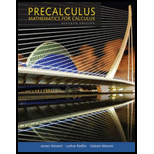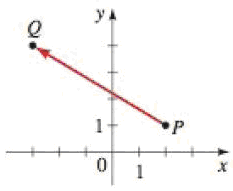Chapter 9.1, Problem 10EPrecalculus: Mathematics for Calcu...

7th Edition
James Stewart + 2 others
ISBN: 9781305071759

Solutions

Chapter
SectionPrecalculus: Mathematics for Calcu...

7th Edition
James Stewart + 2 others
ISBN: 9781305071759
Textbook Problem

Component Form of Vectors Express the vector with initial point P and terminal point Q in component form.10.To determine

To express: The vector with initial point P and terminal point Q into component form.

Explanation

Definition used:

Component form of a vector:

“If a vector v is represented in the plane with initial point P(x1,y1) and terminal point Q(x2,y2), then v=x2x1,y2y1”.

Calculation:

From the given figure, it is observed that the vector has initial point as P(2,1) and terminal point as Q(3,4)

Still sussing out bartleby?

Check out a sample textbook solution.

See a sample solution

The Solution to Your Study Problems

Bartleby provides explanations to thousands of textbook problems written by our experts, many with advanced degrees!

Get Started

Solve the equations in Exercises 126. 2x3x12x+3x+1=0

Finite Mathematics and Applied Calculus (MindTap Course List)

In Exercises 107-120, factor each expression completely. 116. (x + y)2 1

Applied Calculus for the Managerial, Life, and Social Sciences: A Brief Approach

In Problem 1-10, find the real solutions to each quadratic equation.

Mathematical Applications for the Management, Life, and Social Sciences

Evaluate the integral. 11t(1t)2dt

Single Variable Calculus: Early Transcendentals

A normal vector to the plane 2x + 3y + 6z =–20 is:

Study Guide for Stewart's Multivariable Calculus, 8th

Which graph represents y=x+1x

Study Guide for Stewart's Single Variable Calculus: Early Transcendentals, 8th

Find the exact area of the shaded segment. _

Elementary Geometry for College Students